# Samplers#

When rendering an image, Mitsuba 3 has to solve a high-dimensional integration problem that involves the geometry, materials, lights, and sensors that make up the scene. Because of the mathematical complexity of these integrals, it is generally impossible to solve them analytically — instead, they are solved numerically by evaluating the function to be integrated at a large number of different positions referred to as samples. Sample generators are an essential ingredient to this process: they produce points in a (hypothetical) infinite dimensional hypercube $$[0, 1]^{\infty}$$ that constitute the canonical representation of these samples.

To do its work, a rendering algorithm, or integrator, will send many queries to the sample generator. Generally, it will request subsequent 1D or 2D components of this infinite-dimensional point and map them into a more convenient space (for instance, positions on surfaces). This allows it to construct light paths to eventually evaluate the flow of light through the scene.

## Independent sampler (independent)#

Parameter

Type

Description

Flags

sample_count

integer

Number of samples per pixel (Default: 4)

seed

integer

Seed offset (Default: 0)

The independent sampler produces a stream of independent and uniformly distributed pseudorandom numbers. Internally, it relies on the PCG32 random number generator by Melissa O’Neill.

This is the most basic sample generator; because no precautions are taken to avoid sample clumping, images produced using this plugin will usually take longer to converge. Looking at the figures below where samples are projected onto a 2D unit square, we see that there are both regions that don’t receive many samples (i.e. we don’t know much about the behavior of the function there), and regions where many samples are very close together (which likely have very similar values), which will result in higher variance in the rendered image.

This sampler is initialized using a deterministic procedure, which means that subsequent runs of Mitsuba should create the same image. In practice, when rendering with multiple threads and/or machines, this is not true anymore, since the ordering of samples is influenced by the operating system scheduler. Although these should be absolutely negligible, with relative errors on the order of the machine epsilon ($$6\cdot 10^{-8}$$) in single precision.1024 samples projected onto the first two dimensions.#64 samples projected onto the first two dimensions and their projection on both 1D axis (top and right plot).#
<sampler type="independent">
<integer name="sample_count" value="64"/>
</sampler>


## Stratified sampler (stratified)#

Parameter

Type

Description

Flags

sample_count

integer

Number of samples per pixel. This number should be a square number (Default: 4)

seed

integer

Seed offset (Default: 0)

jitter

boolean

Adds additional random jitter withing the stratum (Default: True)

The stratified sample generator divides the domain into a discrete number of strata and produces a sample within each one of them. This generally leads to less sample clumping when compared to the independent sampler, as well as better convergence.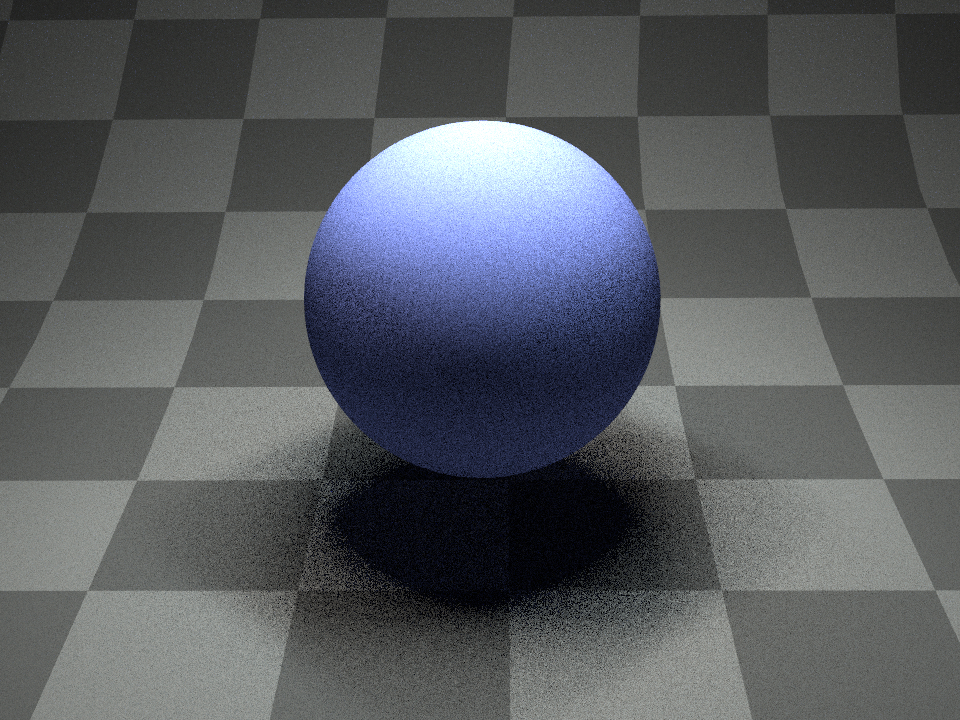Independent sampler - 16 samples per pixel#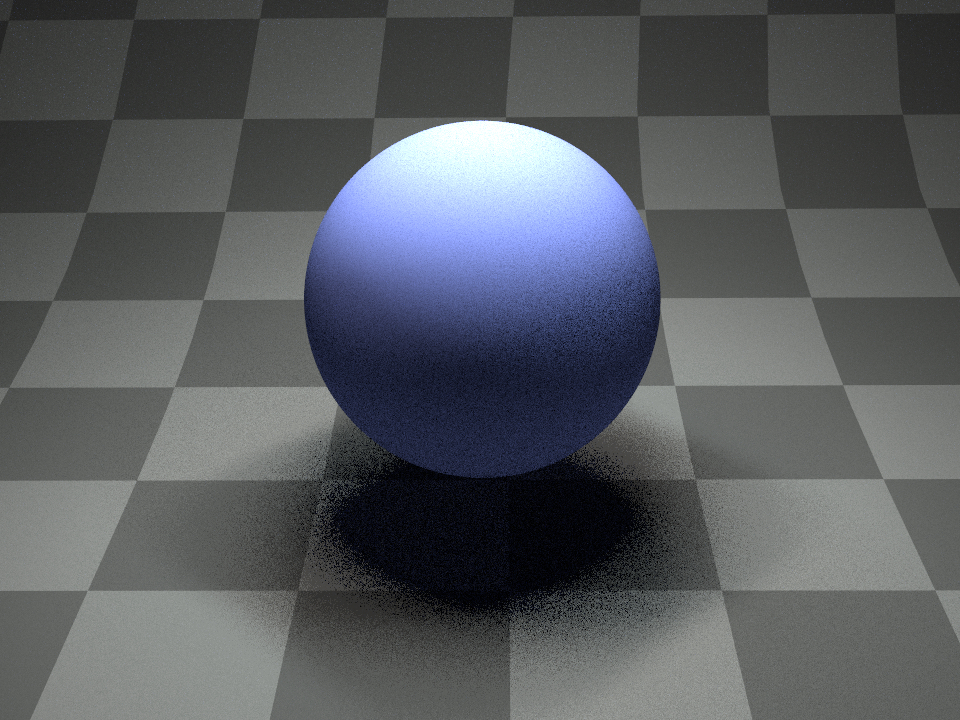Stratified sampler - 16 samples per pixel#1024 samples projected onto the first two dimensions which are well distributed if we compare to the independent sampler.#64 samples projected in 2D and on both 1D axis (top and right plot). Every strata contains a single sample creating a good distribution when projected in 2D. Projections on both 1D axis still exhibit sample clumping which will result in higher variance, for instance when sampling a thin stretched rectangular area light.#
<sampler type="stratified">
<integer name="sample_count" value="64"/>
</sampler>


## Correlated Multi-Jittered sampler (multijitter)#

Parameter

Type

Description

Flags

sample_count

integer

Number of samples per pixel. The sampler may internally choose to slightly increase this value to create a subdivision into strata that has an aspect ratio close to one. (Default: 4)

seed

integer

Seed offset (Default: 0)

jitter

boolean

Adds additional random jitter withing the substratum (Default: True)

This plugin implements the methods introduced in Pixar’s tech memo [Ken13].

Unlike the previously described stratified sampler, multi-jittered sample patterns produce samples that are well stratified in 2D but also well stratified when projected onto one dimension. This can greatly reduce the variance of a Monte-Carlo estimator when the function to evaluate exhibits more variation along one axis of the sampling domain than the other.

This sampler achieves this by first placing samples in a canonical arrangement that is stratified in both 2D and 1D. It then shuffles the x-coordinate of the samples in every columns and the y-coordinate in every rows. Fortunately, this process doesn’t break the 2D and 1D stratification. Kensler’s method further reduces sample clumpiness by correlating the shuffling applied to the columns and the rows.Independent sampler - 16 samples per pixel#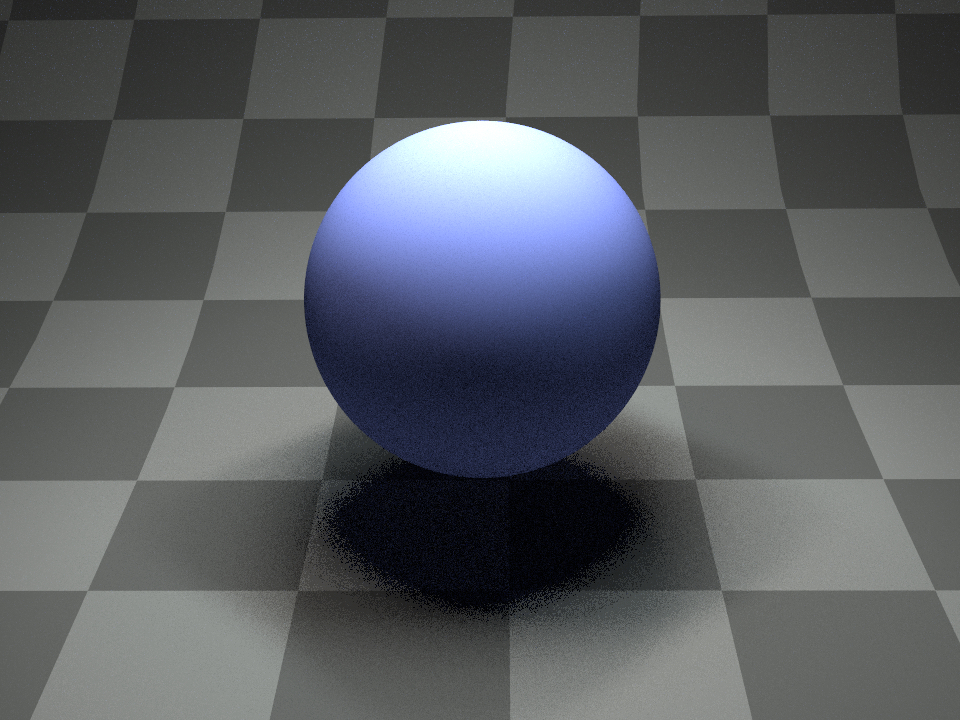Correlated Multi-Jittered sampler - 16 samples per pixel#1024 samples projected onto the first two dimensions.#64 samples projected onto the first two dimensions and their projection on both 1D axis (top and right plot). As expected, the samples are well stratified both in 2D and 1D.#
<sampler type="multijitter">
<integer name="sample_count" value="64"/>
</sampler>


## Orthogonal Array sampler (orthogonal)#

Parameter

Type

Description

Flags

sample_count

integer

Number of samples per pixel. This value has to be the square of a prime number. (Default: 4)

strength

integer

Orthogonal array’s strength (Default: 2)

seed

integer

Seed offset (Default: 0)

jitter

boolean

Adds additional random jitter withing the substratum (Default: True)

This plugin implements the Orthogonal Array sampler generator introduced by Jarosz et al. [JEK+19]. It generalizes correlated multi-jittered sampling to higher dimensions by using orthogonal arrays (OAs). An OA of strength $$s$$ has the property that projecting the generated samples to any combination of $$s$$ dimensions will always result in a well stratified pattern. In other words, when $$s=2$$ (default value), the high-dimensional samples are simultaneously stratified in all 2D projections as if they had been produced by correlated multi-jittered sampling. By construction, samples produced by this generator are also well stratified when projected on both 1D axis.

This sampler supports OA of strength other than 2, although this isn’t recommended as the stratification of 2D projections of those samples wouldn’t be ensured anymore.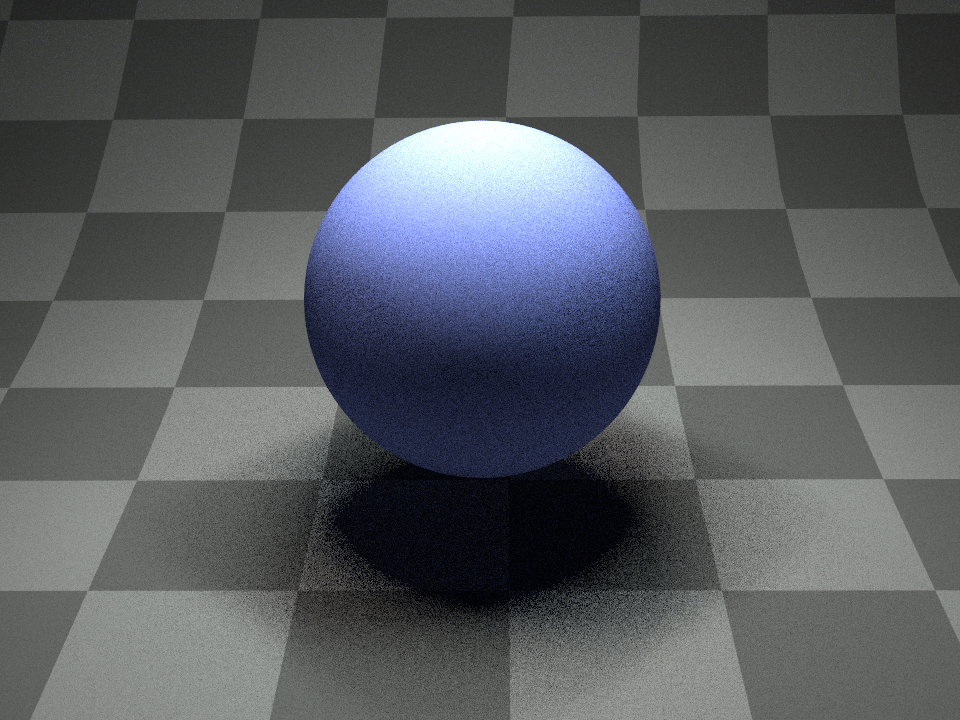Independent sampler - 25 samples per pixel#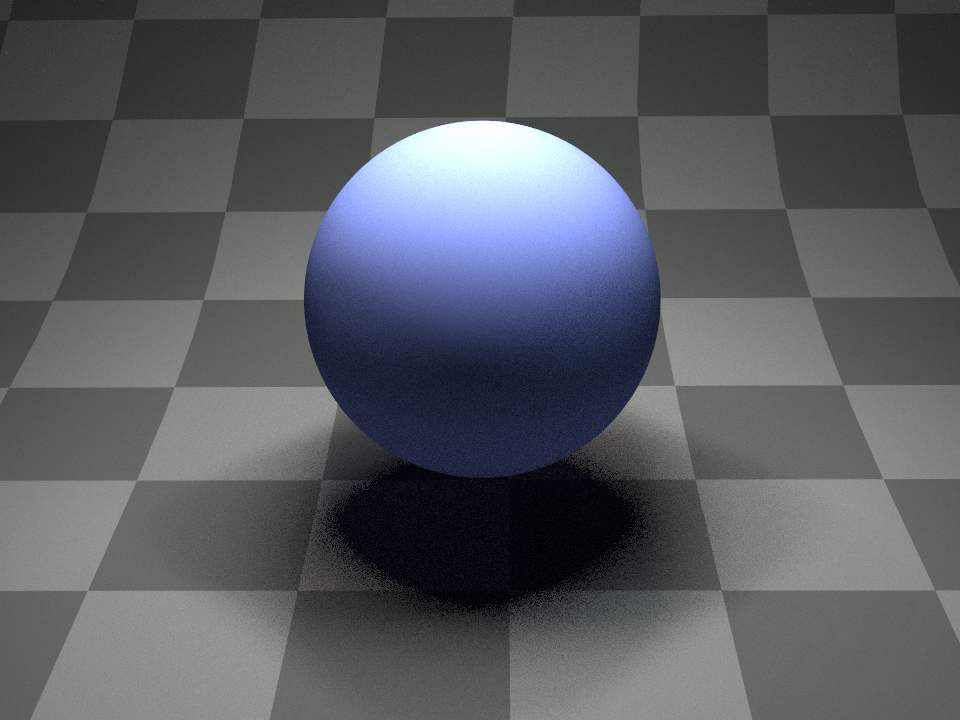Orthogonal Array sampler - 25 samples per pixel#1369 samples projected onto the first two dimensions.#49 samples projected onto the first two dimensions and their projection on both 1D axis (top and right plot). The pattern is well stratified in both 2D and 1D projections. This is true for every pair of dimensions of the high-dimensional samples.#
<sampler type="orthogonal">
<integer name="sample_count" value="4"/>
</sampler>


## Low discrepancy sampler (ldsampler)#

This plugin implements a simple hybrid sampler that combines aspects of a Quasi-Monte Carlo sequence with a pseudorandom number generator based on a technique proposed by Kollig and Keller [KK02]. It is a good and fast general-purpose sample generator. Other QMC samplers exist that can generate even better distributed samples, but this comes at a higher cost in terms of performance. This plugin is based on Mitsuba 1’s default sampler (also called ldsampler).

Roughly, the idea of this sampler is that all of the individual 2D sample dimensions are first filled using the same (0, 2)-sequence, which is then randomly scrambled and permuted using a shuffle network. The name of this plugin stems from the fact that, by construction, (0, 2)-sequences achieve a low star discrepancy, which is a quality criterion on their spatial distribution.Independent sampler - 16 samples per pixel#Low-discrepancy sampler - 16 samples per pixel#1024 samples projected onto the first two dimensions.#A projection of the first 64 samples onto the first two dimensions and their projection on both 1D axis (top and right plot). The 1D stratification is perfect as this sampler doesn’t add additional random perturbation to the sample positions.#1024 samples projected onto the 32th and 33th dimensions, which look almost identical. However, note that the points have been scrambled to reduce correlations between dimensions.#A projection of the first 64 samples onto the 32th and 33th dimensions.#
<sampler type="ldsampler">
<integer name="sample_count" value="64"/>
</sampler>